# E Water Quality Value Graphs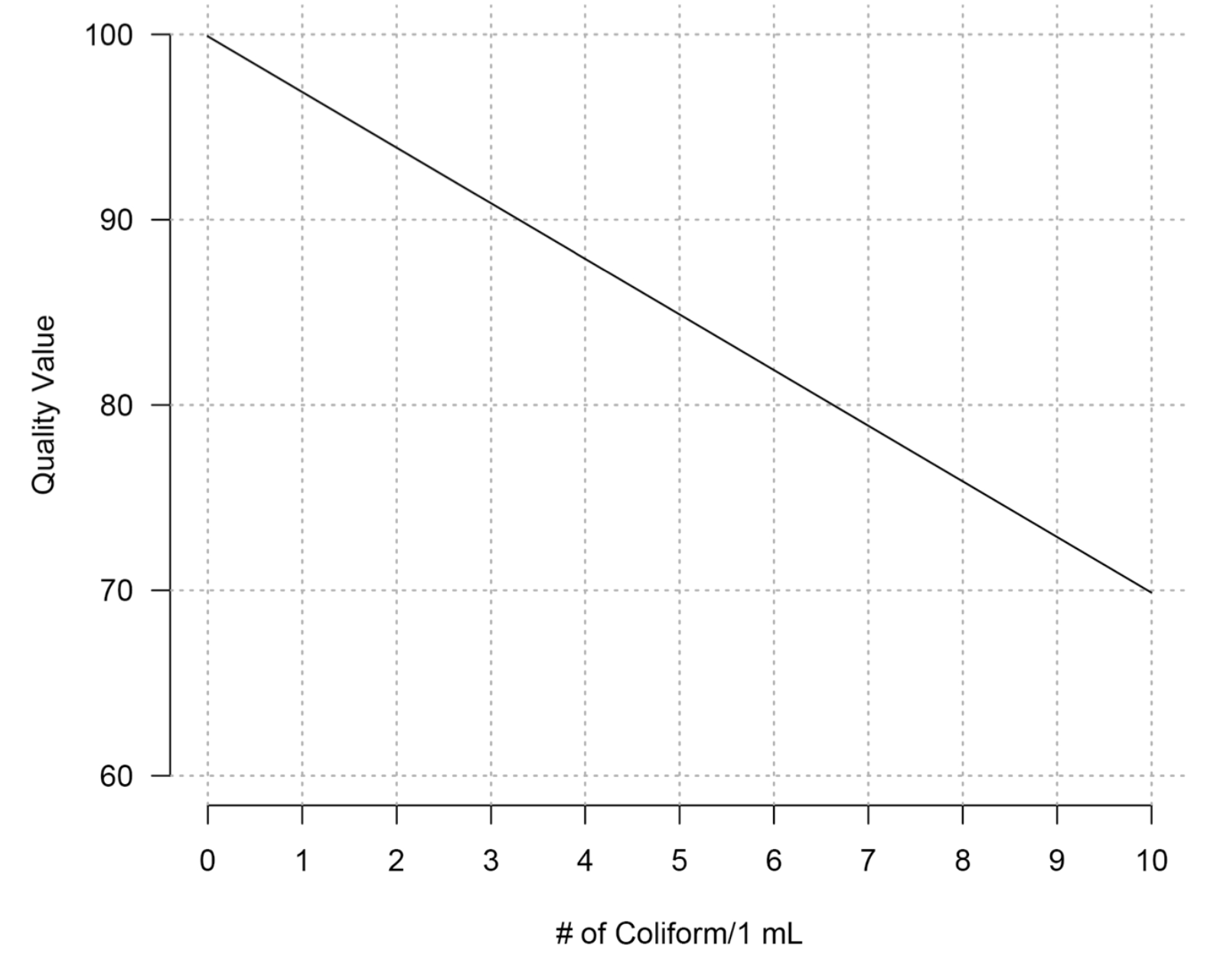Figure E.1: Coliform quality values. Note: extrapolate if necessary, but the quality value cannot be lower than zero.

If the number of coliform colonies that you counted are greater than 10, you can use the following relationship:
$$y = -3x + 100$$

where:

• $$y$$ is the quality value
• $$x$$ is the number of coliform colonies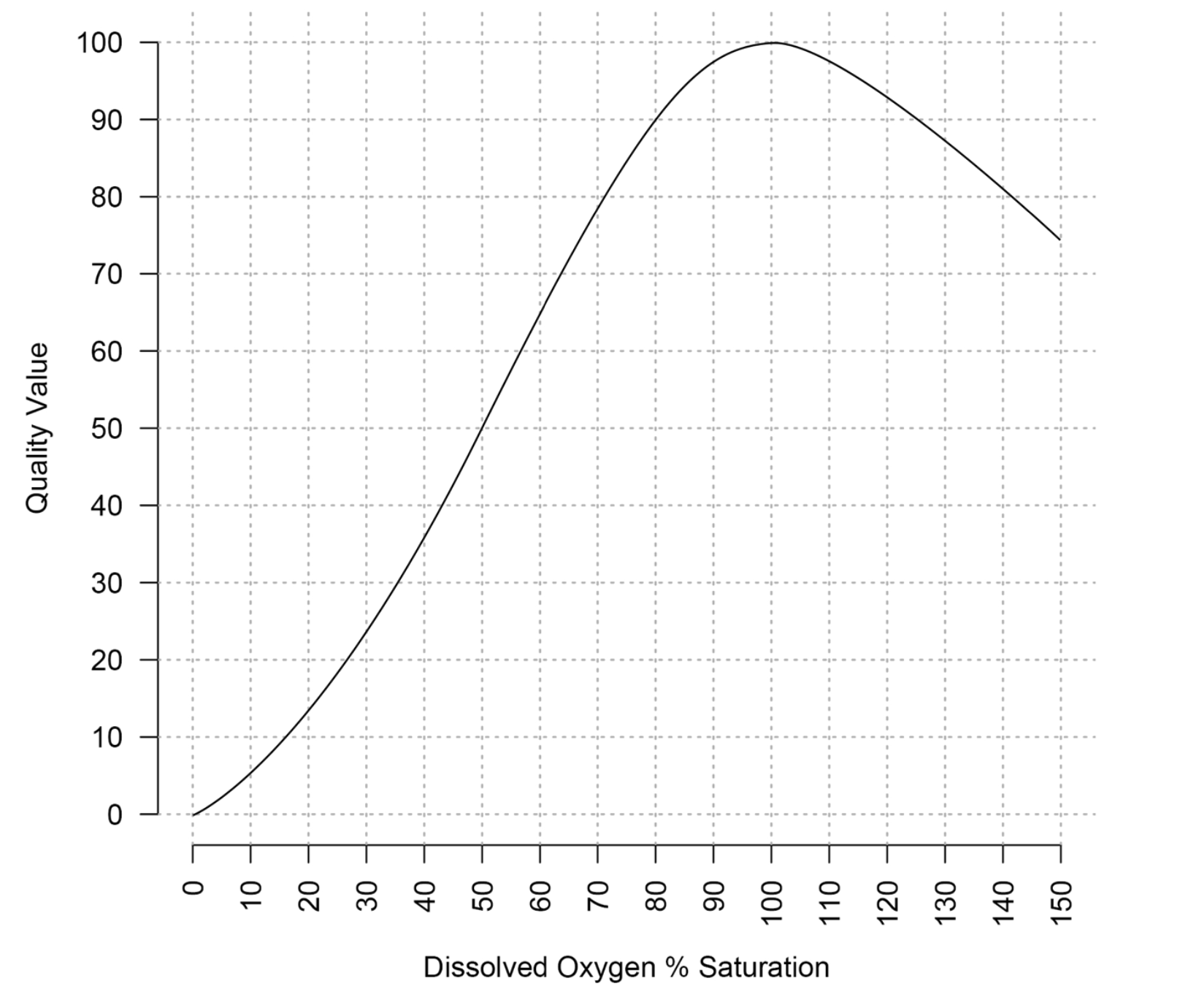Figure E.2: Dissolved oxygen percent saturation quality values.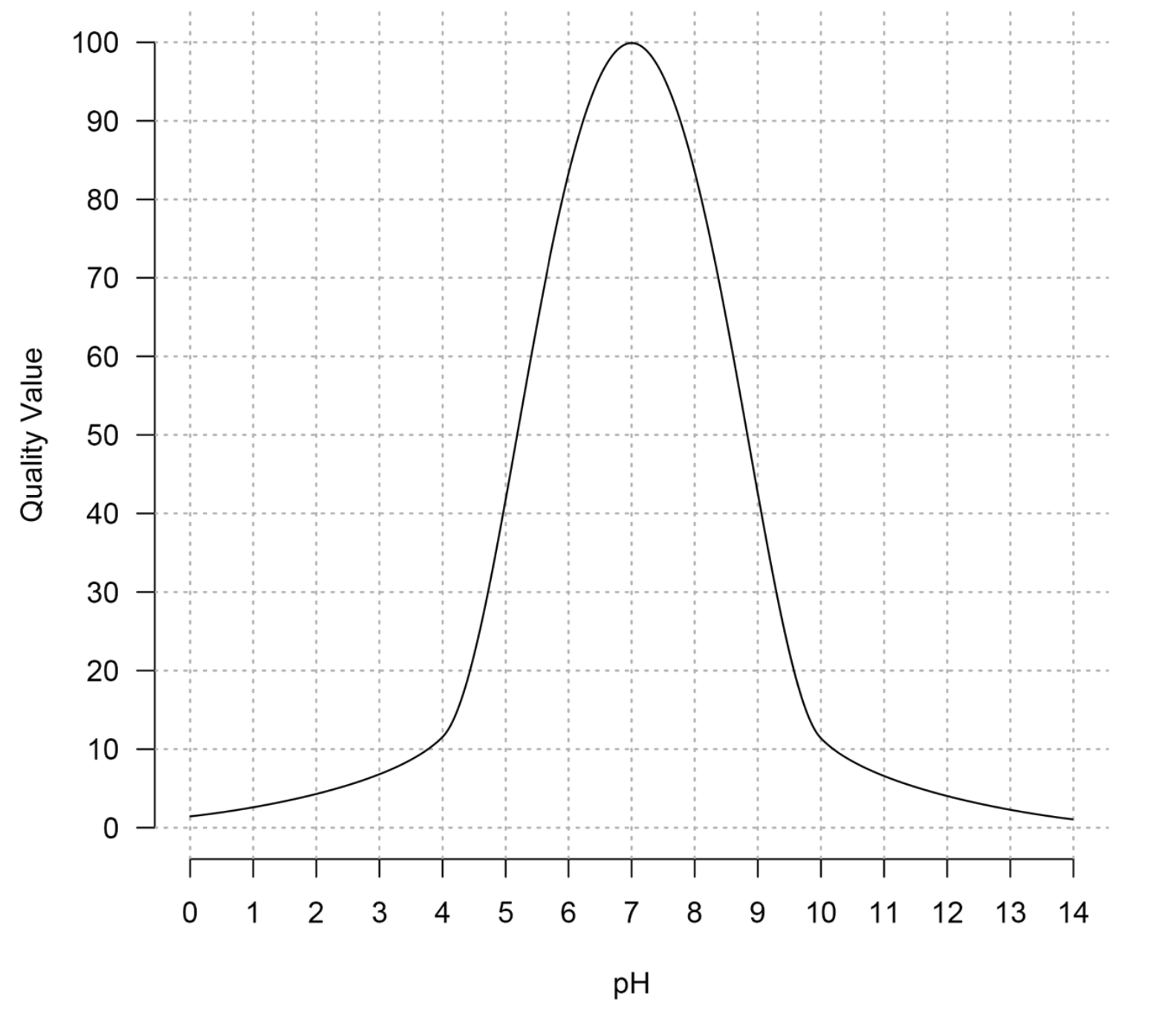Figure E.3: pH quality values.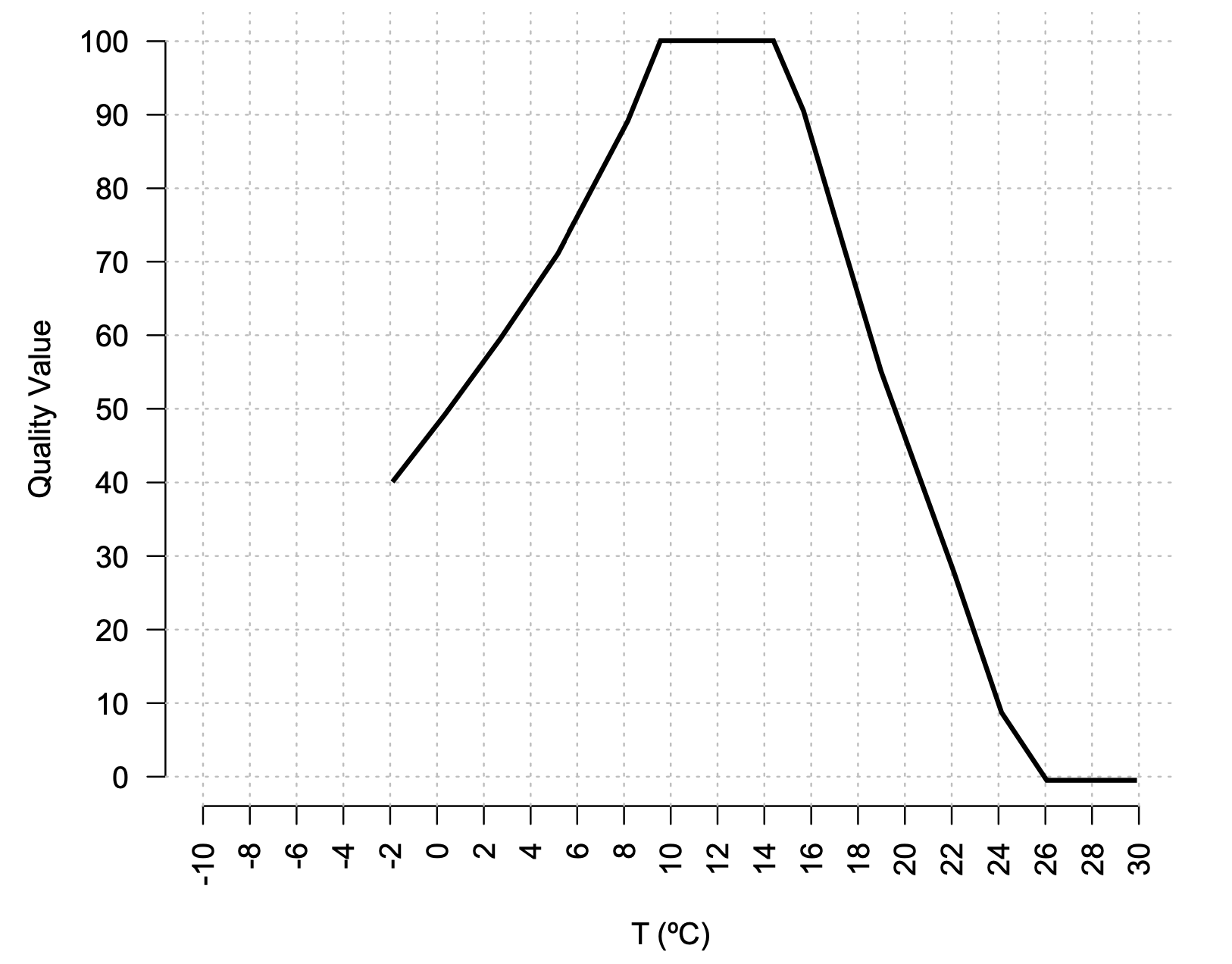Figure E.4: Water temperature quality values.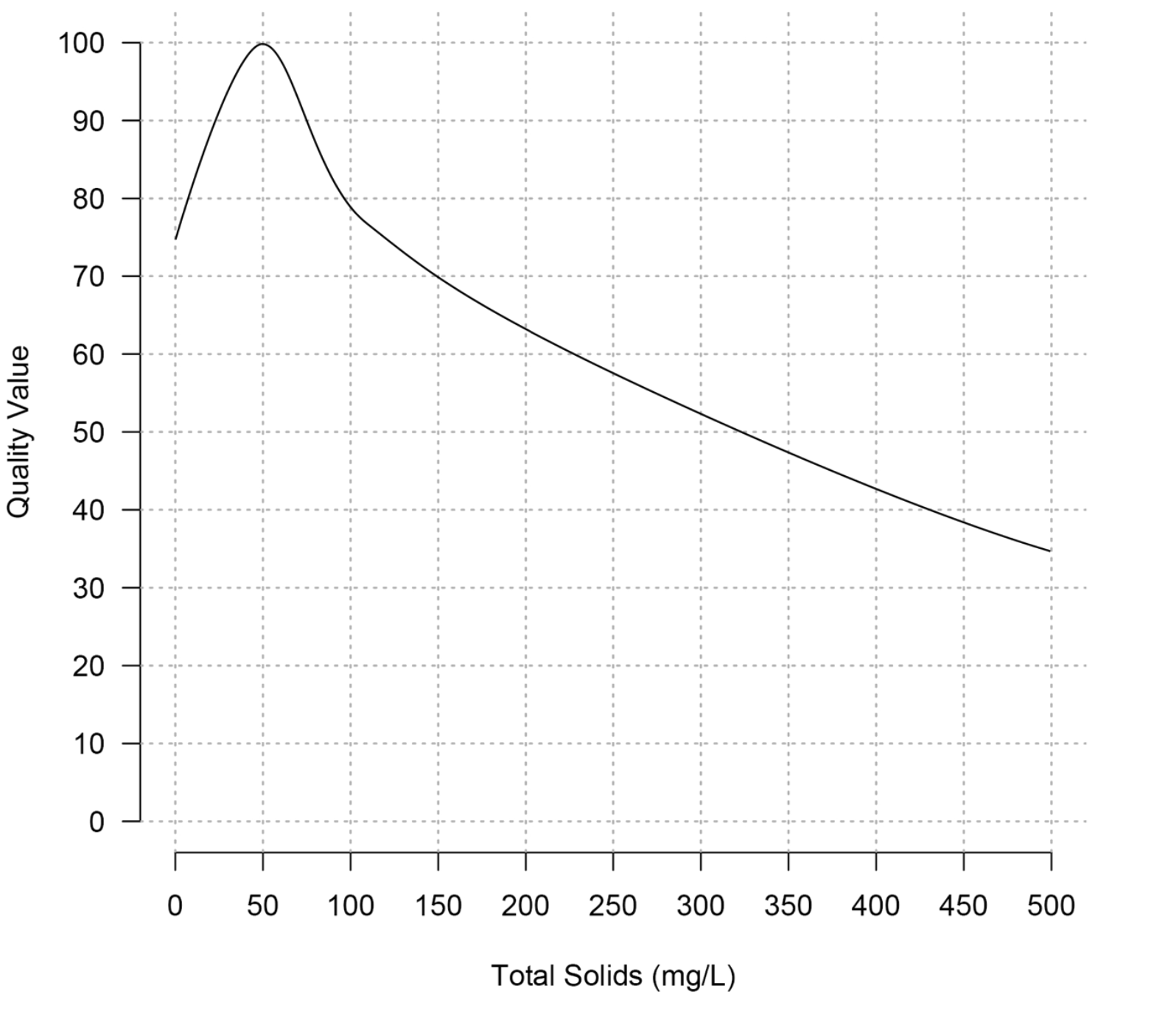Figure E.5: Total solids quality values.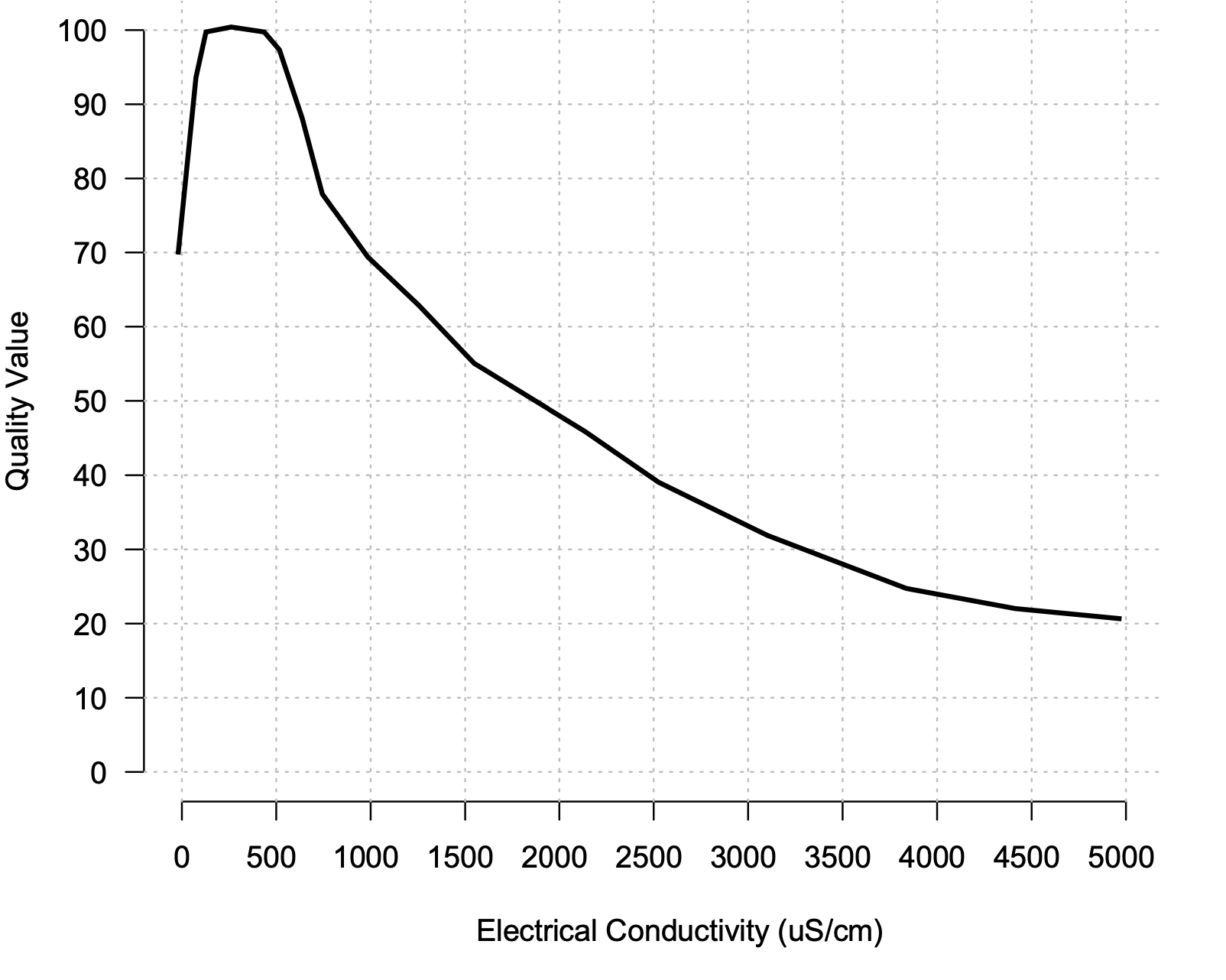Figure E.6: Electrical conductivity quality values.